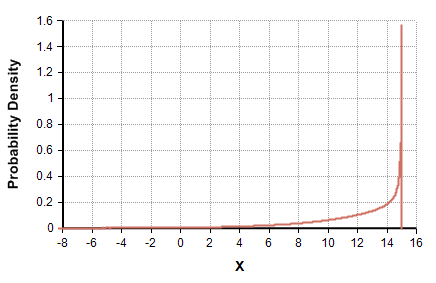# Smooth Fractile

(diff) ← Older revision | Latest revision (diff) | Newer revision → (diff)

## Smooth_Fractile(fract, F)

Given a set of fractiles, this function returns a smooth distribution with tails having the indicated fractiles. The fractiles to use must be specified in «F», each value being between 0 and 1, and the fractile values must be in «fract».

Declaration:

Smooth_Fractile(fract: Ascending[F]; F: positive ascending Index)

## Library

Distribution Variations library (Distribution Variations.ana)

## Example

To specify a distribution having a P10, P50 and P90 of 7, 13, and 15, set:

F := [0.1, 0.5, 0.9]
fract := Table(F) (7, 13, 15)

and call

Smooth_Fractile(fract, F)